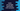# Kotlin groupingBy explanation with example## Introduction :

groupingBy function is used to group items and apply one operation using a keySelector function. It returns one Grouping object that we can use to call its other methods. In this post, I will quickly show you how to use groupingBy with examples.

### Example of groupingBy :

As I have mentioned above, groupingBy returns one Grouping object and we can use it to get other information. For example, eachCount method of Grouping converts it to a map of key and count of the items of each key as the value.

``````fun main() {
val userGroup = listOf("Alex", "Albert", "Chandler", "Bob", "Camila", "Diana")

val userGroupMap = userGroup.groupingBy { it.first() }.eachCount()

print(userGroupMap)
}``````

This example will give the below output :

``{A=2, C=2, B=1, D=1}``

The key is the first character of each word in the list. eachCount converts the map to key as the character and value as the number of words starts with that character. For example, we have two words that start with A, so its value is 2.

### Example of groupingBy with reduce :

We can use other methods of Grouping object as well. For example, we can use it with the reduce method as like below :

``````fun main() {
val intGroup = listOf(122, 233, 1234, 11234, 2233, 333, 345, 3567, 45)

val intGroupMap = intGroup.groupingBy { it.toString().first() }.reduce{_, a,b -> maxOf(a,b)}

print(intGroupMap)
}``````

The reduce operation used in this example finds out the largest number from the values. It uses maxOf to find out the max value. The key is the first digit of the number. If we execute this program, it will print the below output :

``{1=11234, 2=2233, 3=3567, 4=45}``

Among numbers starts with 1, 11234 is the largest. Similarly, 2233 is the largest of all starts with 2, etc.